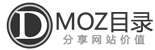### 世界节日大全

• 新年元旦[01.01]
• 腊八节[农历十二月初八]

• 世界湿地日[02.02]
• 国际气象节[02.10]
• 情人节[02.14]
• 除夕[农历十二月三十]
• 春节[农历正月初一]
• 元宵节[农历正月十五]

### 三月

• 全国爱耳日[03.03]
• 妇女节[03.08]
• 植树节[03.12]
• 国际警察日[03.14]
• 国际消费日[03.15]
• 世界森林日[03.21]
• 世界水日[03.22]
• 世界气象日[03.23]
• 世界防治结核病日[03.24]

• 愚人节[04.01]
• 清明节[04.05]
• 世界卫生日[04.07]
• 世界地球日[04.22]

### 五月

• 国际劳动节[05.01]
• 中国青年节[05.04]
• 全国碘缺乏病日[05.05]
• 世界红十字日[05.08]
• 国际护士节[05.12]
• 国际家庭日[05.15]
• 世界电信日[05.17]
• 国际博物馆日[05.18]
• 全国助残日[05.19]
• 全国学生营养日[05.20]
• 国际生物多样性日[05.22]
• 国际牛奶日[05.23]
• 世界无烟日[05.31]
• 端午节[农历五月初五]
• 母亲节[第二星期日]

### 六月

• 国际儿童节[06.01]
• 世界环境日[06.05]
• 全国爱眼日[06.06]
• 端午节[06.15]
• 父亲节[第三个星期日]
• 防治荒漠化和干旱日[06.17]
• 国际奥林匹克日[06.23]
• 全国土地日[06.25]
• 国际反毒品日[06.26]

### 七月

• 香港回归日[07.01]
• 建党日[07.01]
• 抗日战争纪念日[07.07]
• 世界人口日[07.11]

### 八月

• 八一建军节[08.01]
• 七夕情人节[农历七月初七]

### 九月

• 劳动节[09.01]
• 国际扫盲日[09.08]
• 教师节[09.10]
• 国际臭氧层保护日[09.16]
• 国际和平日[09.17]
• 国际爱牙日[09.20]
• 中秋节[农历八月十五]
• 国际聋人节[09.22]
• 世界旅游日[09.27]
• 重阳节[农历九月初九]

### 十月

• 国庆节[10.01]
• 国际音乐节[10.01]
• 国际减轻自然灾害日[10.02]
• 世界动物日[10.04]
• 国际住房日[10.07]
• 全国高血压日[10.08]
• 世界视觉日[10.08]
• 世界邮政日[10.09]
• 世界精神卫生日[10.10]
• 国际盲人节[10.15]
• 世界粮食节[10.16]
• 世界消除贫困日[10.17]
• 世界传统医药日[10.22]
• 联合国日[10.24]
• 万圣节[10.31]

### 十一月

• 中国记者日[11.01]
• 消防宣传日[11.09]
• 世界糖尿病日[11.14]
• 国际大学生节[11.17]
• 感恩节[11.28]

### 十二月

• 世界艾滋病日[12.01]
• 世界残疾人日[12.03]
• 世界足球日[12.09]
• 冬至节[12.22]
• 圣诞节[12.25]